## Method

Introspective Vision for Simultaneous Localization and Mapping [st][lc] [IV-SLAM]
https://github.com/ut-amrl/IV_SLAM

Submitted on 24 Mar. 2021 17:05 by
Sadegh Rabiee (University of Texas at Austin)

 Running time: 0.1 s Environment: GPU @ 2.5 Ghz (C/C++)

 Method Description: IV-SLAM learns a context-aware noise model for the reprojection errors from visual features in an autonomously supervised manner. The learned noise model is then incorporated into the joint maximum likelihood estimation in run-time. This is an implementation of IV-SLAM for ORB-SLAM2. Parameters: ORBextractor.nFeatures: 2000 ORBextractor.scaleFactor: 1.2 ORBextractor.nLevels: 8 Latex Bibtex: @inproceedings{rabiee2020ivslam, title={IV-SLAM: Introspective Vision for Simultaneous Localization and Mapping}, author={Sadegh Rabiee and Joydeep Biswas}, booktitle={Conference on Robot Learning (CoRL)}, year={2020}, }

## Detailed Results

From all test sequences (sequences 11-21), our benchmark computes translational and rotational errors for all possible subsequences of length (5,10,50,100,150,...,400) meters. Our evaluation ranks methods according to the average of those values, where errors are measured in percent (for translation) and in degrees per meter (for rotation). Details for different trajectory lengths and driving speeds can be found in the plots underneath. Furthermore, the first 5 test trajectories and error plots are shown below.

## Test Set Average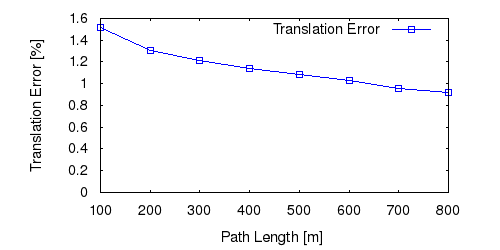This figure as: png eps pdf txt gnuplot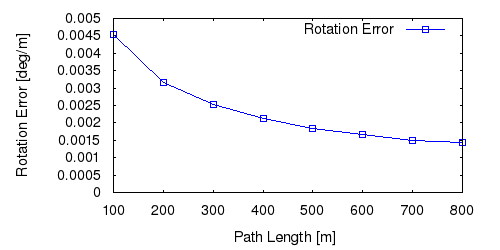This figure as: png eps pdf txt gnuplot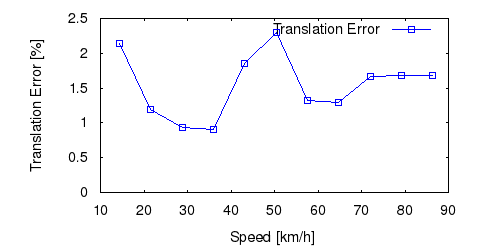This figure as: png eps pdf txt gnuplot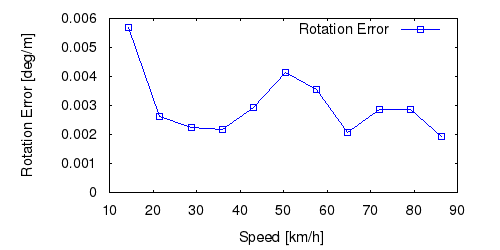This figure as: png eps pdf txt gnuplot

## Sequence 11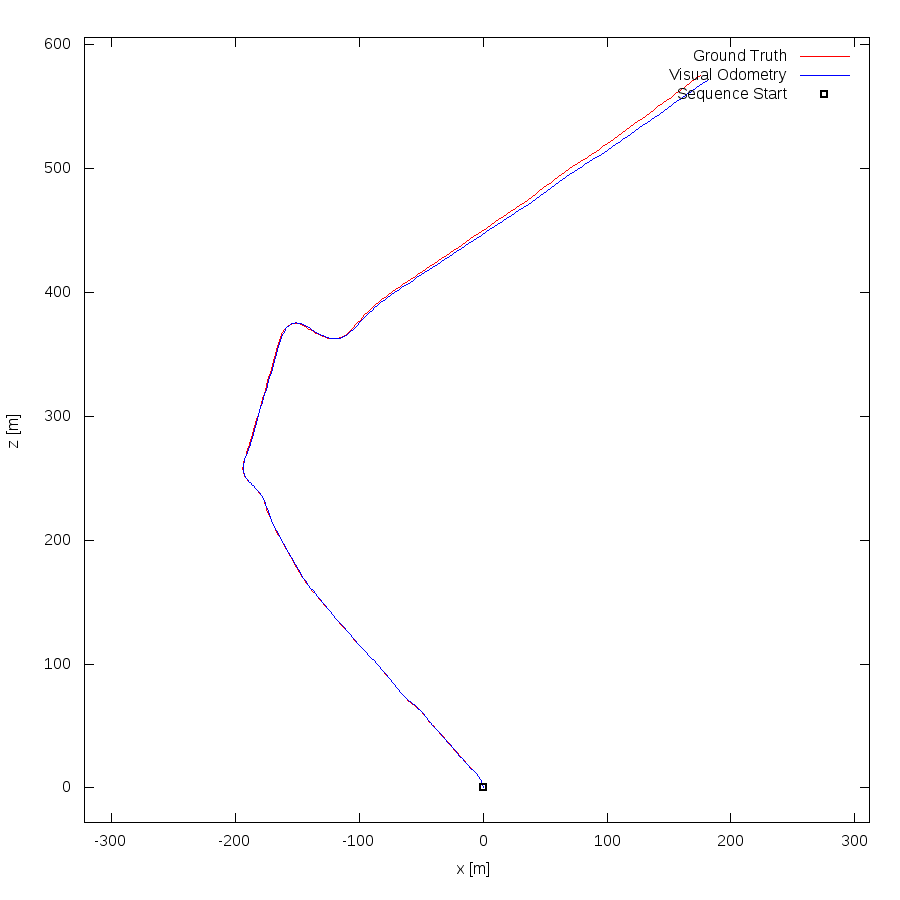This figure as: png eps pdf txt gnuplot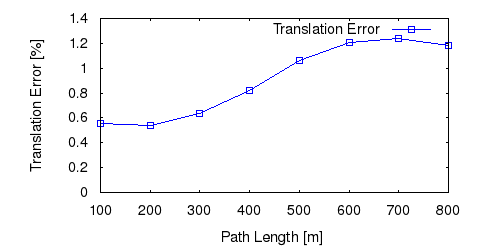This figure as: png eps pdf txt gnuplot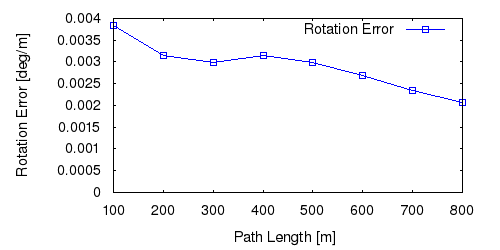This figure as: png eps pdf txt gnuplot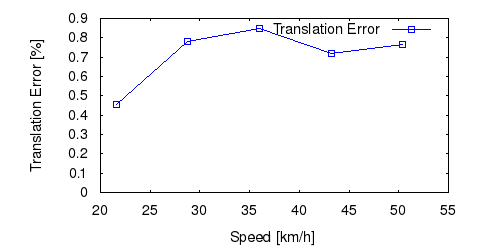This figure as: png eps pdf txt gnuplot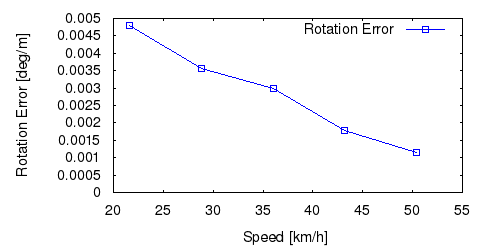This figure as: png eps pdf txt gnuplot

## Sequence 12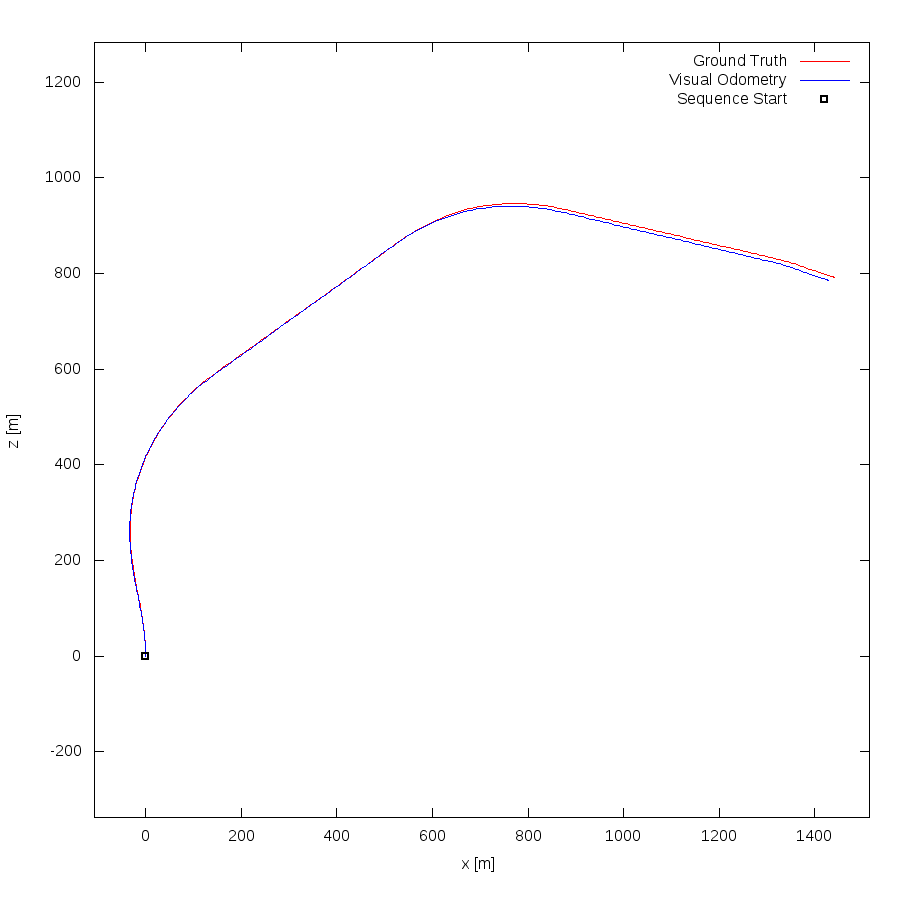This figure as: png eps pdf txt gnuplot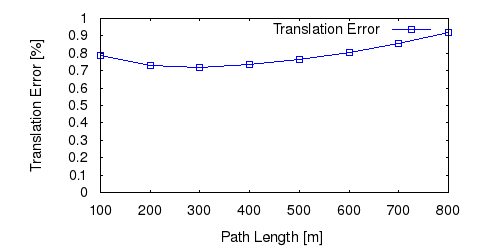This figure as: png eps pdf txt gnuplot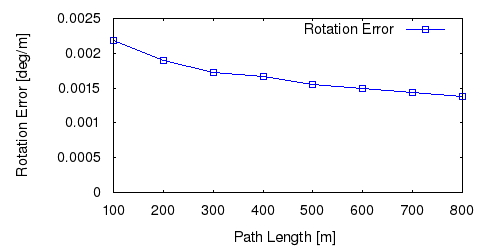This figure as: png eps pdf txt gnuplot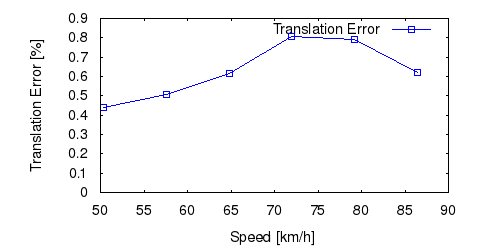This figure as: png eps pdf txt gnuplot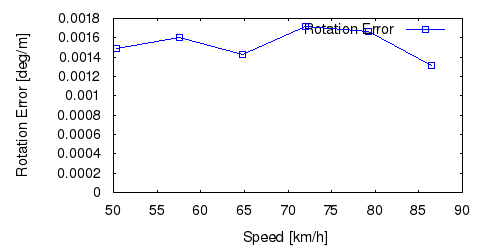This figure as: png eps pdf txt gnuplot

## Sequence 13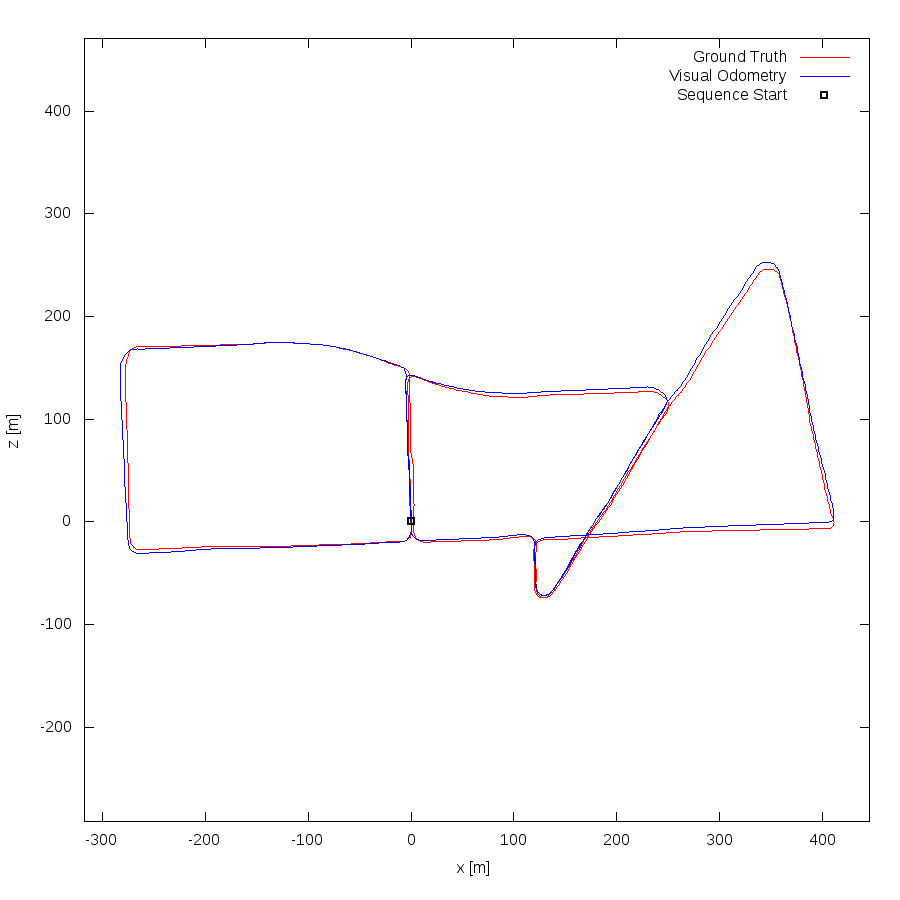This figure as: png eps pdf txt gnuplot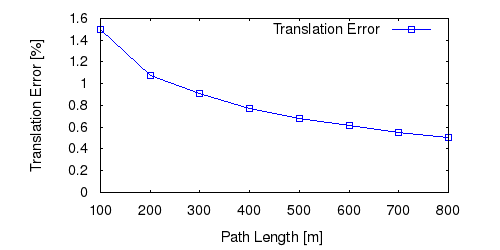This figure as: png eps pdf txt gnuplot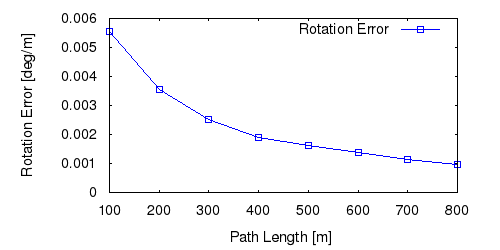This figure as: png eps pdf txt gnuplot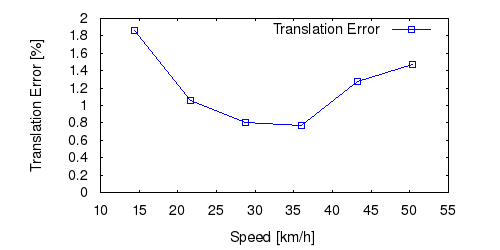This figure as: png eps pdf txt gnuplot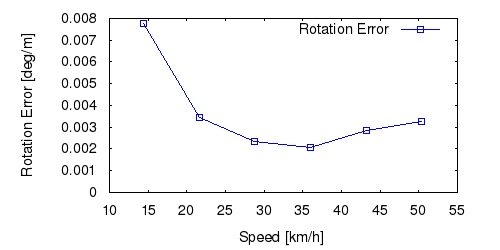This figure as: png eps pdf txt gnuplot

## Sequence 14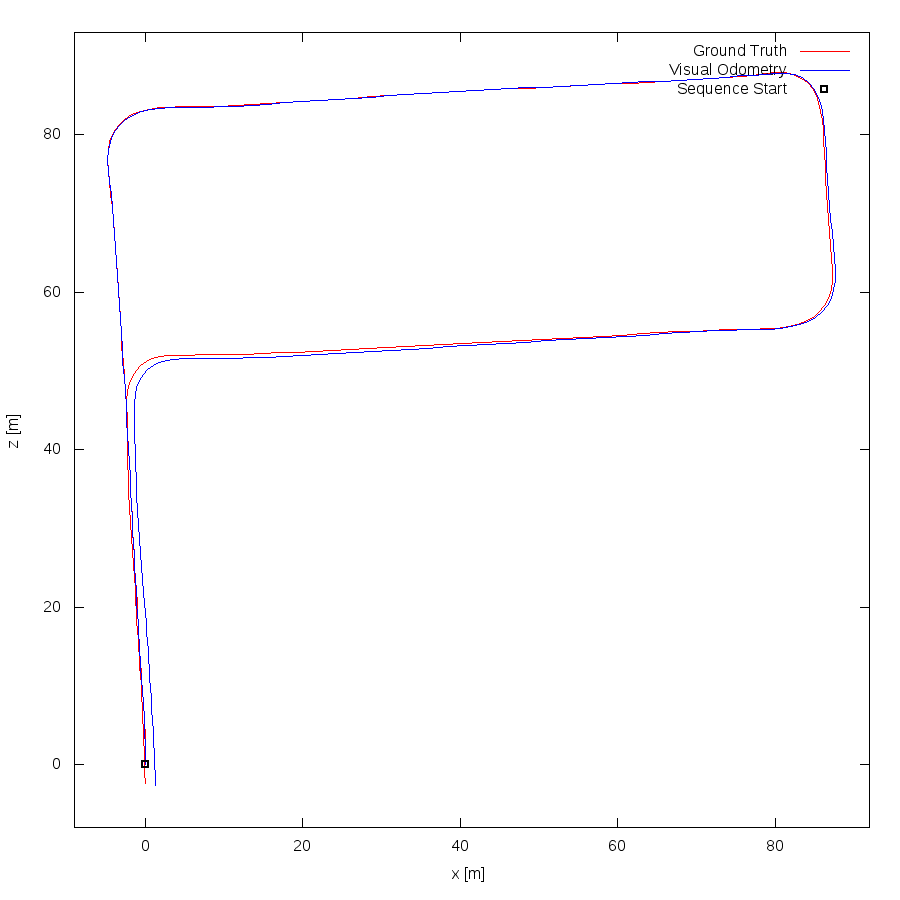This figure as: png eps pdf txt gnuplot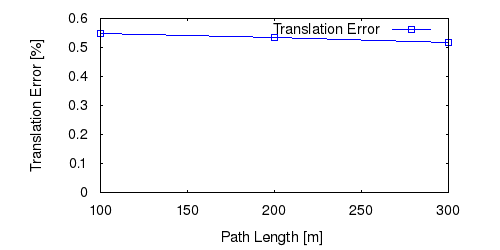This figure as: png eps pdf txt gnuplot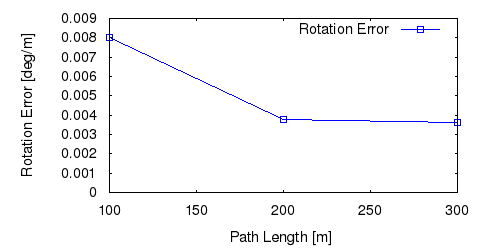This figure as: png eps pdf txt gnuplot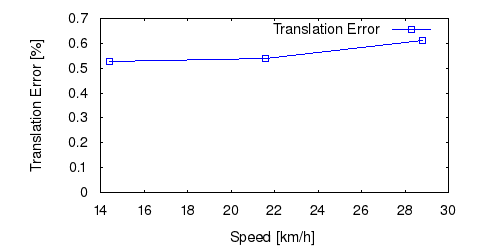This figure as: png eps pdf txt gnuplot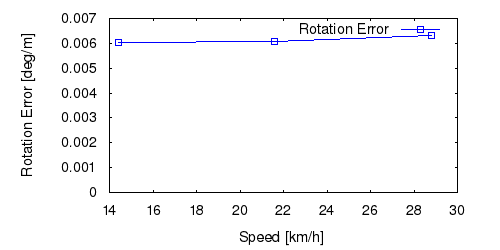This figure as: png eps pdf txt gnuplot

## Sequence 15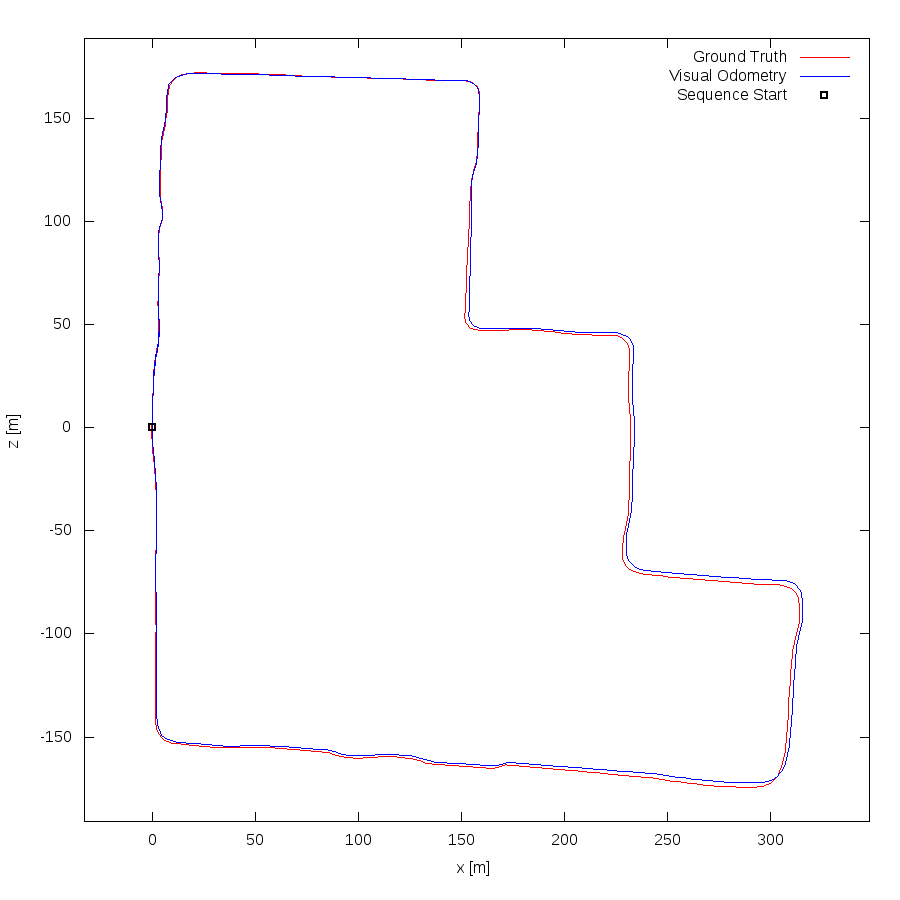This figure as: png eps pdf txt gnuplot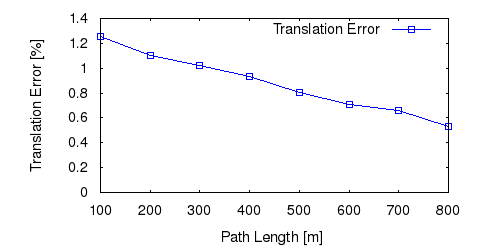This figure as: png eps pdf txt gnuplot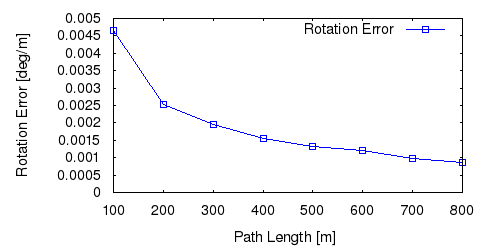This figure as: png eps pdf txt gnuplot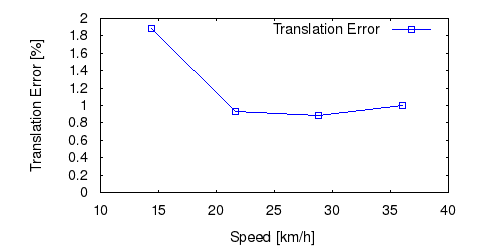This figure as: png eps pdf txt gnuplot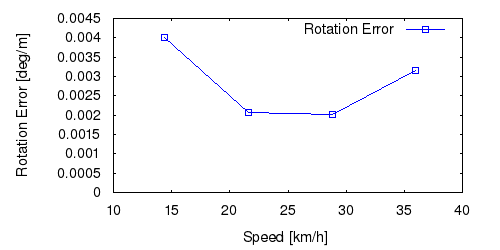This figure as: png eps pdf txt gnuplot